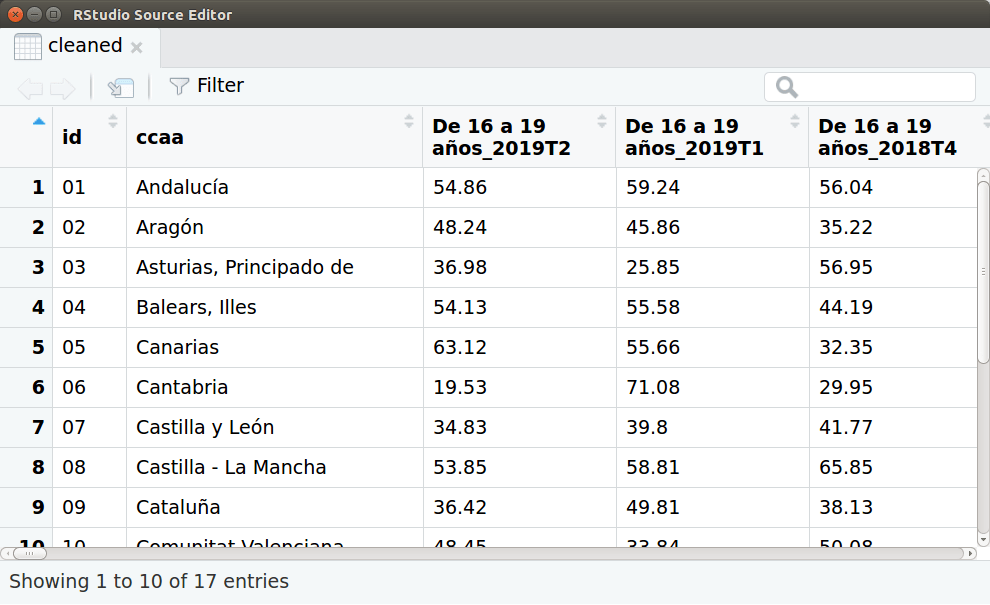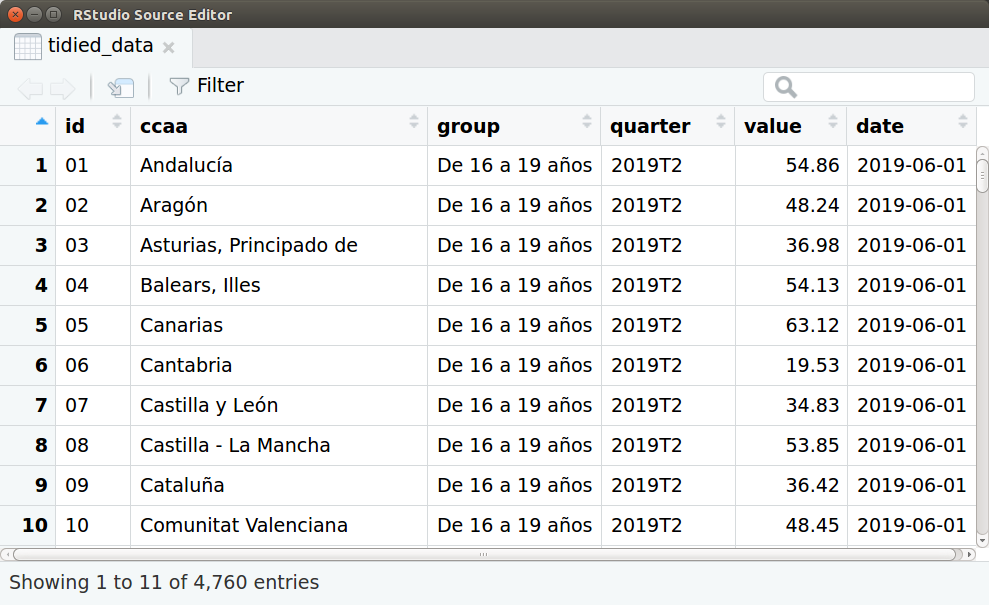# Merge CSV headers in R

R Tidyverse

Usually when you work with data from Public Data Services like INE (Spanish Statistical Office) you have to deal with a Excel files with a non usable format: metadata in the first rows, notes at the bottom, empty columns, empty rows, etc. You have to do a small data processing in order to start working.

Everyone knows the benefits of the CSV format, but precisely these metadata are there for one reason: unlike CSV where we only have the data itself, if we open this file within a few months this metadata will let you know exactly what data it contains or where it comes from. For this reason, I think that sometimes it is not a bad idea that the first phase of working with data is reading directly from an Excel format (instead of CSV).

For these cases I usually use R due to its reproducible workflow and of course because of the whole universe of packages, specially Tidyverse, an opinionated collection of R packages designed for data science.

Well, days ago I had to deal with a dataset that had a format with a problem I didn’t face before, it had a double header like the ones shown in the following picture:Capture from the Spanish Statistical Office site. Unemployment rate by ages and year quarters.

I spent hours searching the web looking for a solution but I didn’t find any. I finally opted for create a function to clean this dataset. My goal was to merge both rows and ended up with a column names as `Menores de 25 años_2019T2`, `Menores de 25 años_2019T1` or `Menores de 25 años_2018T4`, i.e. combine the two rows with an underscore. I will show step by step a case in which this function could be used. If you prefer, you can go directly to the full script. First of all, I read a xlsx file with the amazing `read_xlsx` from Tidyverse and skip the first 6 lines.

``````library(tidyverse)
library(ggplot2)
library(janitor)

filePath <- "data/4247.xlsx"

csv <- read_xlsx(filePath, skip = 6)
``````

The function itself:

``````# This function fills empty cells with previous values to the right and then combine them with the row above

# store the row
row <- colnames(data)

# Get unique values, the ones will be repeated
types <- row[is.na(as.numeric(gsub("\\.", "", row)))]

# Create an index that will be incremented across the vector 'types'
z <- 1

# Store the first as the default one
type <- types[z]

# Iterate through colnames
for(i in 1:length(row)){
variable <- row[i]

# Assume the work starts at the second column
if (i!=1) {
# combine with the previous row
row[i] <- paste(type, data[1, ][i], sep = "_")

# If current value is equal to the second one from unique values it updates the default value
if (z + 1 <= length(types) & variable == types[z + 1]) {
z <- z + 1
type <- types[z]
row[i] <- paste(type, data[1, ][i], sep = "_")
}
}

}
return(row)
}
``````

I am completely sure that there must be a cleaner way or an R package that solves this problem but I have been unable to find it. Basically, what `combineHeaders` does is to store in the variable `types` those column names of the first row in a vector (discarding those column names that are numbers, as R / RStudio puts by default). Then iterates through all the column names in the first row (the original one from which we have extracted `types`), creates a default index (variable `z`) initialized to `1` and creates a variable called `type` that points to the first element of `types` through `z` value. In this iteration, it assigns the first element of the `types` vector (the value of the `type`) to the first elements. If a column name is equal to the next `types` element, it increases the value of `z` by one and updates `type`.

Then I assign the vector result of the funcion as the new csv header and apply some dplyr’s magic pipes for cleaning the data, renaming the first column and create a couple of variables from the first column.

``````# Assign the new headers from combineHeaders

cleaned <- csv %>%
remove_empty('cols') %>%
remove_empty('rows') %>%
slice(3:19) %>%
rename(
ccaa = 1
) %>%
mutate(
id = substr(ccaa, 0, 2),
ccaa = substring(ccaa, 3)
) %>%
select(id, everything(), -matches("Menores de 25 años"), -matches("25 y más años"))

``````Capture of the data-frame ‘cleaned’.

Then I convert all the data-frame to long format, divide the previous headers into two new variables using `separate` and parse temporal variables to a date format:

``````tidied_data <- gather(cleaned, group, value, 3:length(colnames(cleaned))) %>%
separate(group, c('group', 'quarter'), sep = '_') %>%
mutate(
value = as.numeric(value),
date = as.Date(paste(substring(quarter, 1, 4), as.integer(substring(quarter, 6, 7)) * 3, 1, sep = "-"))
)
``````Capture of the data-frame ‘tidied_data’.

And finally a plot using ggplot. The code:

``````ggplot(data=tidied_data, aes(x = date, y = value)) +
geom_line(aes(color = group)) +
scale_color_manual(values = c("#966bff", "#FF6AD5", "#ffde8b", "#20de8b")) + # Color scale by vapeplot
facet_wrap( ~ ccaa, ncol = 4) +
ggtitle("Unemployment rates by age groups and regions") +
theme_minimal() +
theme(axis.title.x=element_blank(),
axis.ticks.x=element_blank(),
axis.title.y=element_blank(),
axis.ticks.y=element_blank(),
plot.margin = unit(c(0.5,0.5,0.5,0.5), "cm"))
``````

And the result: